# Electric Flux through a circle

Davidllerenav
Homework Statement:
A point charge q is located at the origin. Consider the electric field
flux through a circle a distance from q, subtending an angle 2θ, as
shown in Fig. 1.36. Since there are no charges except at the origin,
any surface that is bounded by the circle and that stays to the right
of the origin must contain the same flux. (Why?) Calculate this
flux by taking the surface to be:

(a) the flat disk bounded by the circle;
(b) the spherical cap (with the sphere centered at the origin)
bounded by the circle.
Relevant Equations:
Gauss Law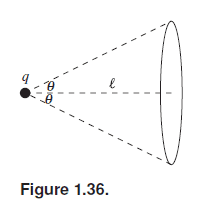Hi! My main problem is that I don't understand what the problem is telling me. What does it mean that the surface is a flast disc bounded by the circle? Is the Gauss surface the disc? Does that mean that inside the circle in the figure, there is a disc?

Can you give me some guidance on how to begin solving the problem?

•Delta2

Homework Helper
Gold Member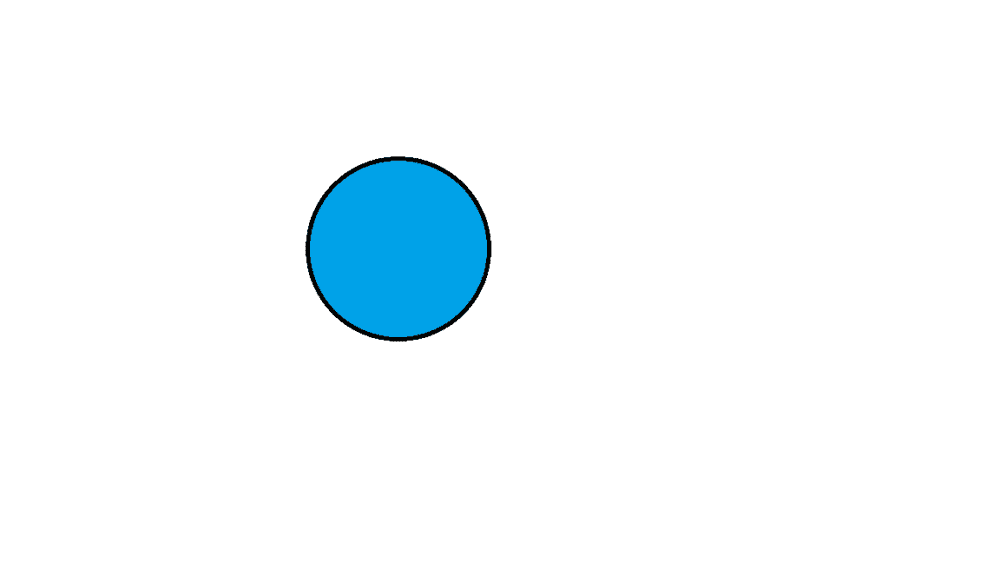The black (and curved) line is the circle. The blue area is the disc bounded by the circle.
A circular disc is a surface but is not a gaussian surface. A gaussian surface is a closed surface that encloses a 3D volume pretty much like the surface of a sphere encloses the volume of the sphere. if you can imagine that the blue disc blows and stretches (while the boundary of the disc (the black circle) remains unstretched) towards yourself then this streched and blown disc would make a gaussian surface( I know its kind of hard to explain with words without making 3D schemes).
To start solving a) you must setup a surface integral $$\int\int_A\vec{E}\cdot\vec{dS}$$ where E is the expression for the electric field in the various points of the circular disk and A is the set of points of space that makeup the circular disk. Then you got to calculate the integral.

Davidllerenav
View attachment 249473
The black (and curved) line is the circle. The blue area is the disc bounded by the circle.
A circular disc is a surface but is not a gaussian surface. A gaussian surface is a closed surface that encloses a 3D volume pretty much like the surface of a sphere encloses the volume of the sphere. if you can imagine that the blue disc blows and stretches (while the boundary of the disc (the black circle) remains unstretched) towards yourself then this streched and blown disc would make a gaussian surface( I know its kind of hard to explain with words without making 3D schemes).
To start solving a) you must setup a surface integral $$\int\int_A\vec{E}\cdot\vec{dS}$$ where E is the expression for the electric field in the various points of the circular disk and A is the set of points of space that makeup the circular disk. Then you got to calculate the integral.
I understand, thanks. I think I made it, is this correct?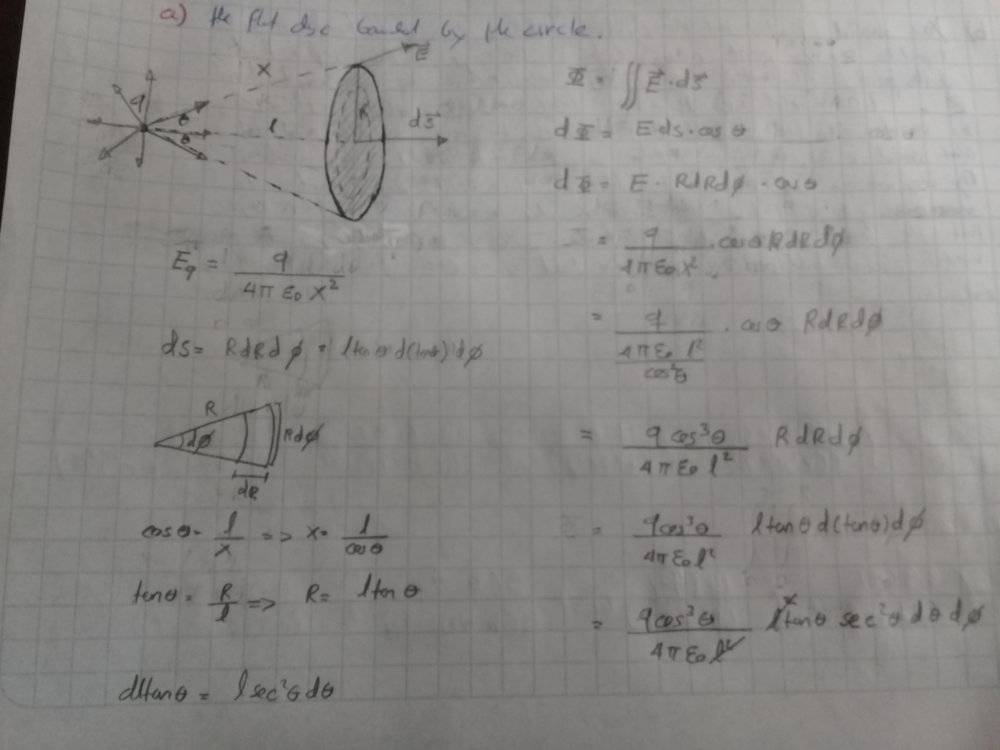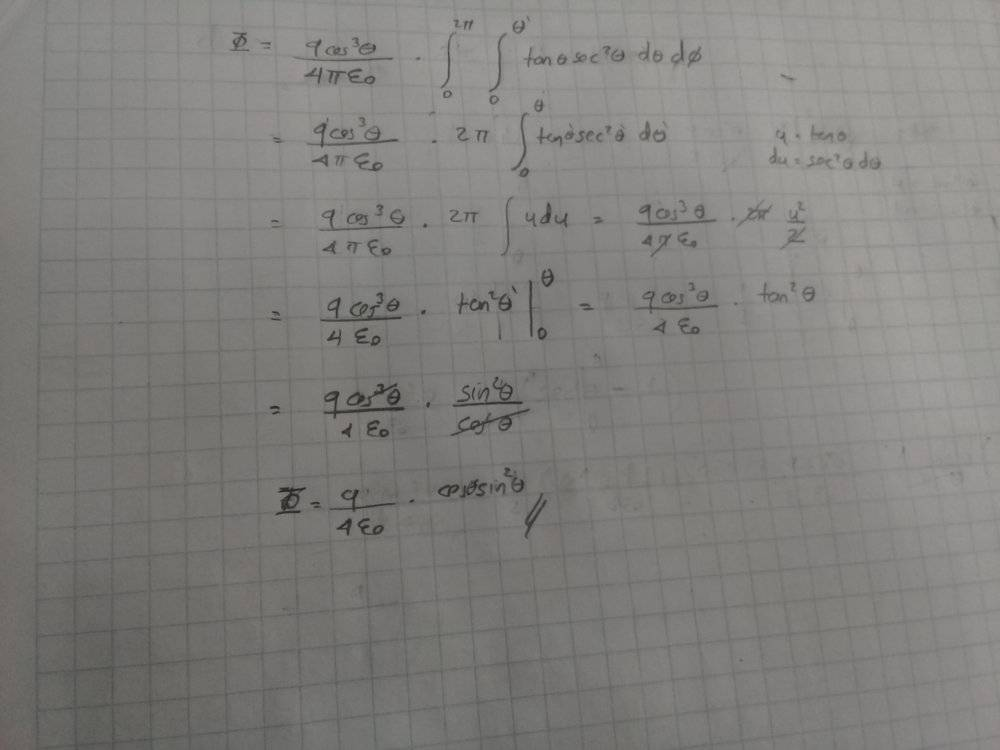Homework Helper
Gold Member
Work at image1 looks good.

But I don't understand why in your subsequent work in image2 you move the ##\cos^3\theta## term out of the integral...

Davidllerenav
Work at image1 looks good.

But I don't understand why in your subsequent work in image2 you move the ##\cos^3\theta## term out of the integral...
I didn't notice, sorry. I corrected and end up with this, I think it is correct.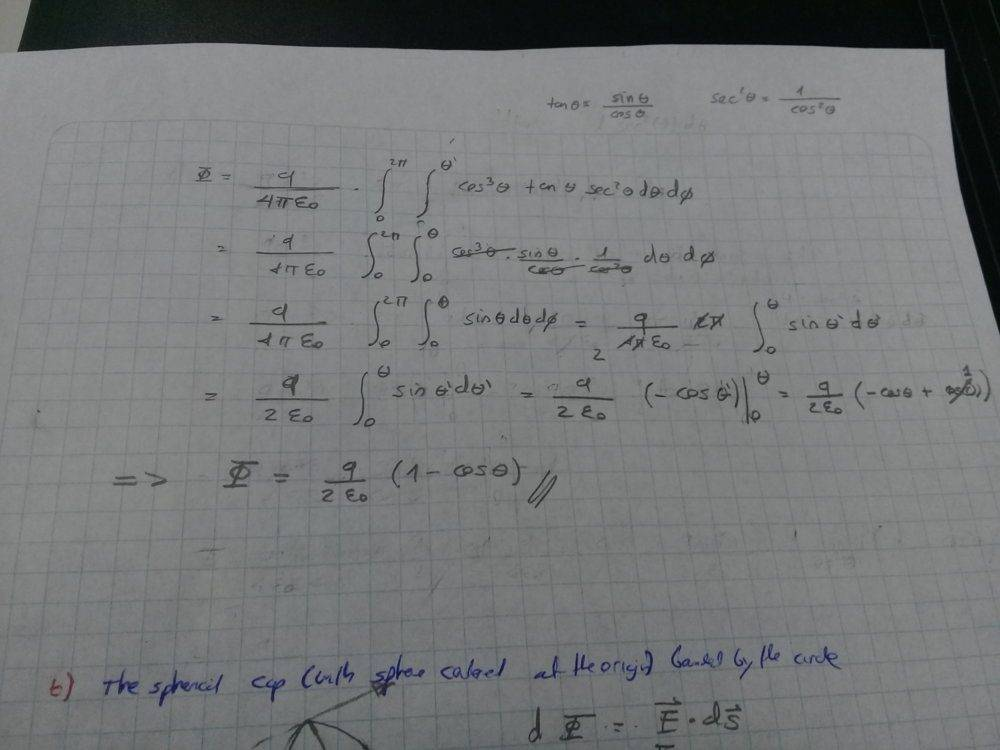Davidllerenav
@Delta2 Is it correct? Can you help me with part b?

Homework Helper
Gold Member
Yes I think it is correct.

For b) use a spherical coordinate system such that the inclination (or polar) angle will be the ##\theta## of figure 1.36 (we basically work in the same coordinate system as in image 1, regarding ##\phi## and ##\theta##, and ##R## now is the radius of the sphere centered at origin). The surface element of the spherical cap is ##dS=R^2\sin\theta d\theta d\phi## in that system. The normal to the surface element of the spherical cap will be always in the same direction as the direction of the electric field in that surface element. So the dot product simplifies to $$\vec{E}\cdot d\vec{S}=|\vec{E}||d\vec{S}|=|\frac{q}{4\pi\epsilon_0R^2}||R^2\sin\theta| d\theta d\phi$$. So all you have to calculate is the integral of the above.

Last edited:
Davidllerenav
Yes I think it is correct.

For b) use a spherical coordinate system such that the inclination (or polar) angle will be the ##\theta## of figure 1.36. In the case of the spherical cap, the normal to the surface element of the spherical cap will be always in the same direction as the direction of the electric field in that surface element. So the dot product simplifies to ##\vec{E}\cdot d\vec{S}=|\vec{E}||d\vec{S}|=|\frac{q}{4\pi\epsilon_0R^2}||R^2\sin\theta| d\theta d\phi##. So all you have to calculate is the integral of the above.
I see, thank you! You've helped me a lot.

•Delta2